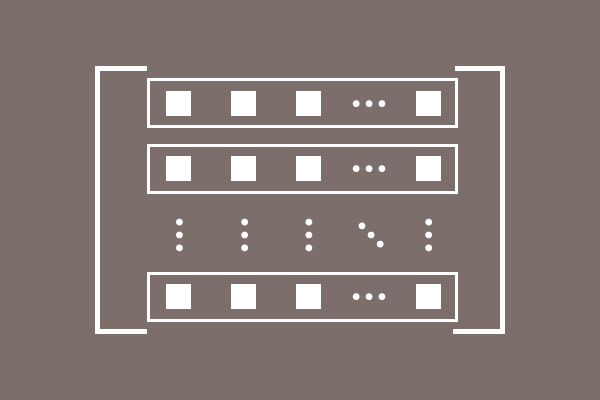# Row of a Matrix

A horizontal line of elements in a matrix is called row of a matrix.The space inside a matrix is divided as number of rows to place elements equally in each row. Every two elements in a row is separated by equal space.

Similarly, an equal space between every two rows in a matrix is maintained to avoid mixing elements of every two rows.

#### Example

$\begin{bmatrix} -4 & 1 & 0 & 9 \\ 7 & 3 & -8 & 5\\ 2 & -5 & 1 & 0\\ \end{bmatrix}$

1. The horizontal line of elements $-4,$ $1,$ $0$ and $9$ is called the first row of matrix.
2. The horizontal line of elements $7,$ $3,$ $-8$ and $5$ is called the second row of matrix.
3. The horizontal line of elements $2,$ $-5,$ $1$ and $0$ is called the third row of matrix.
Latest Math Topics
Jun 26, 2023
Jun 23, 2023

Latest Math Problems
Jul 01, 2023
Jun 25, 2023
###### Math Questions

The math problems with solutions to learn how to solve a problem.

Learn solutions

Practice now

###### Math Videos

The math videos tutorials with visual graphics to learn every concept.

Watch now

###### Subscribe us

Get the latest math updates from the Math Doubts by subscribing us.